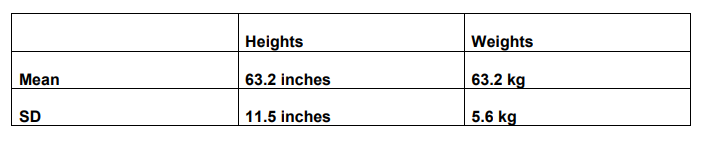Deepak Scored 45->99%ile with Bounce Back Crack Course. You can do it too!

# The mean and variance of the heights and weights of the students of a class are given below:

Question:

The mean and variance of the heights and weights of the students of a class are given below:Which shows more variability, heights or weights?

Solution:

In case of heights,

Mean $=63.2$ inches and $S D=11.5$ inches

So, the coefficient of variation,

$\mathrm{CV}=\frac{\mathrm{SD}}{\text { Mean }} \times 100$

$\Rightarrow \mathrm{CV}=\frac{11.5}{63.2} \times 100=18.196$

In case of weights,

Mean $=63.2$ inches and $S D=5.6$ inches.

So, the coefficient of variation,

$\mathrm{CV}=\frac{\mathrm{SD}}{\text { Mean }} \times 100$

$\Rightarrow \mathrm{CV}=\frac{5.6}{63.2} \times 100=8.86$

$\mathrm{CV}$ of heights $>\mathrm{CV}$ of weights